﻿ Utilities for Spatial Route Analysis 空间路径分析工具 – 王灿的个人网站

## Utilities for Spatial Route Analysis 空间路径分析工具

1. 这是一个完全免费的工具。
This is a completely free tool.

2. 我不是专业码农，因此不能保证功能不出现问题。如果你在使用中发现任何bug，请和我联系，除了用户电脑本身的问题以外，绝大多数的问题都可以得到及时解决。
It is hard to get rid of all bugs, and I cannot make guarantee for the functionality of the tool. If you find any bug when using it, please contact with me. I promise that most of the problems (expect those caused only by your computer) could be solved in time.

3. 本工具基于Python 3.6开发，不需要安装运行环境，直接下载、解压后找到exe文件就可以用，坏处是打包后的文件有点大。
This tool is developed based on Python 3.6. Compared with Matlab-based tools, python-based tools do not need additional installation of Runtime and thus could be directly executed by double-clicking .exe file, however, they seems to be much larger in file size.

4. 路径分析的内容很广，本工具目前只是中间版本，希望在以后根据需要不断增加新的功能。
There are so much to do in spatial route analysis. Consequently, this tool is still under developing, and I hope that new methods would be included upon demanding.

Option 1. Download the executable (packed in zip file, named ‘UtilitiesForSpatialRouteAnalysis.exe’) and directly use it without needs to install python.

Option 2. Those who have already installed python3 could use following zip file, which contains a py file. Please note that this py file would have to import several modules, including pandas, numpy, tkinter, pickle, sklearn, apyori.

python源代码 (python code for this tool)

# 功能介绍 Introduction of Functionality

File 文件

In this tool, a route is a sequence of spaces or places an individual has visited successively. When importing route data by ‘Read CSV Data’, users need to prepare a csv file as follows. The first column is the indices of individuals, and the routes start from the second column. For example, the route of individual 1 is ‘A→B→C→D’, and the route of individual 2 is ‘B→C→D’. A/B/C/D/… are the name of different places. Since the routes do not have the same length, we do not cut them to certain length when reading the data, all valid inputs from the second column and afterwards would be recorded to routes. So when a route ends, please leave the following columns empty and do not add anything, including numbers, ‘end’, ‘over’, or any placeholders, they would be mistakenly viewed as new places.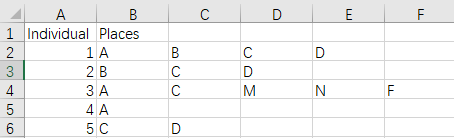Such data could be acquired by questionnaire, GPS, mobile phone big data, etc. This tool provides several interesting methods for this kind of data, including clustering of routes, association among places, and route predicting. Hopefully it will bring convenience for the researchers.

Clustering 聚类

Route clustering is to find several modes from hundreds and thousands of observed routes. Furthermore, we hope that each mode has a representative route, so we could extract typical routes. Since route data is not structured, it is not fit for many traditional clustering algorithms. To solve this problem, we firstly measure the similarity among each pair of routes by running ‘Analyze Route Similarity’, and the output is a N by N similarity matrix, where N is the number of observed routes. We could easily get mean similarity for each route by running ‘Mean Similarity with All Routes’, obviously, the route with larger average similarity would be more typical, However, there might  be a route which is able to represent a friction of routes, but has quite low similarity with other routes, as a result, its average similarity with all routes is not as high as expected. Therefore, in order to find different modes, we need to run clustering analysis (‘Affinity Propagation Clustering’) based on similarity matrix. The output is a table of typical routes, the first column is the indices of individuals, the second column is the typical routes, and the third column is the number of routes represented by the typical routes, or saying, the size of the corresponding clusters.

Association 关联

Routes connect different places, so we could analyze association among places via route data. The simplest association is co-occurrence of two places in a single route. We could run ‘Co-occurrence Matrix’ to get a M by M matrix, where M is the number of places. The values of this matrix represent the number of individuals who have visited both place A and place B. Going a step further, we could image a space network, whose nodes are places and whose connections are co-occurrence relationship. Based on this network, we could run ‘Core/Periphery: Coreness’ to estimate coreness of each place, which reflects the role of the place in the network. The place with larger coreness is expected to have more coupling effect with other places in the network. If you are pretty interested in the relationship of specific two places, you can run ‘Probabilities Concerning Two Places’. By setting one place (Y) as the target place, and another place (X) as the condition place, this tool would calculate several related probabilities, such as the percentage of people also visit Y among those visit X. From these probabilities, you might find potential stimulating or competing relationship. Finally, this tool provides ‘Apriori’ algorithm, which is used to extract rules like ‘if A (or A1, A2, …), then B’, given certain thresholds.

Prediction 预测

The essential task of prediction is to set up a model, which should be able to calculate the probability of any route. We believe that the input data, no matter big data or small data, is just a sample from population, consequently, it is not a satisfactory way that all calculation of probabilities are purely relying on the counts and percentages directly derived from observed data. A better choice is to set up a model behind the data and let the model output probabilities. The mode we used is recurrent neural network, a deep learning approach which has proved to be quite successful in a similar area of predicting language probabilities. As a deep learning algorithm, it need as many data as possible. Users could estimate a RNN model by running ‘Recurrent Neural Network’, and then run ‘Route Probability’ to calculate the probability of an arbitrary route, no matter whether it is included in the sample. Users could also run ‘Step Probability’ to calculate the probability of choosing the last place of a route as the next destination, given that the individual has visited previous places of the route. Finally, users could run ‘Beam Search for Typical Route’ to find a route with the largest probability and thus quite typical.

# 视频示例 Video Demo

The video below briefly introduce the functions of this tool.

The video below focus on how different preference settings affect the result of route clustering.

# 算法 Algorithms

(1) 路径相似度 Route Similarity

Route similarity is measured by Levenstein Ratio, which is often used in NLP for measuring similarity of strings.

Levenstein距离（或称编辑距离）是将一个字符串转化为另一个字符串所需要的最小编辑次数，包括增加字符、替换字符、删除字符。例如，下图中，从字符串“ABCDEFG”转化为字符串“BCD”，需要删除最开始的“A”和最后面的“EFG”，因此有4个删除操作，两个字符串之间的Levenstein距离为4。这是个非常简单的例子，也有些例子没那么直观，例如：从字符串”ABCDEFG”转化为“FABDE”，需要在头部增加“F”，然后删除中间的“C”和尾部的“FG”，共4个操作（1个增加操作+3个删除操作），Levenstein距离也是4。幸运的是，Levenstein距离的计算有非常简洁高效的算法，不管这种转化是否直观，都很容易实现。可以想像，两个字符串的Levenstein距离越大，它们越不相似。
Levenstein Distance (LD, or Edit Distance) is the minimum operations needed to convert from one string to another. The types of operations include insertion, removal, and substitution. For instance, if we want to convert from ‘ABCDEFG’ to ‘BCD’, we just need to delete ‘A’ and ‘EFG’, the 4 operations of removal define a LD of 4 between two strings. For a more complex example, we want to convert from ‘ABCDEFG’ to ‘FABDE’, we need to add a ‘F’ at the beginning of the string, and then delete ‘C’ in the middle and ‘FG’ at the end. LD is also 4, for 1 insertion and 3 removal. Fortunately, there is an efficient algorithm for LD, which makes the calculation very easy. Obviously, the larger the LD between 2 strings, the less similar they are from each other.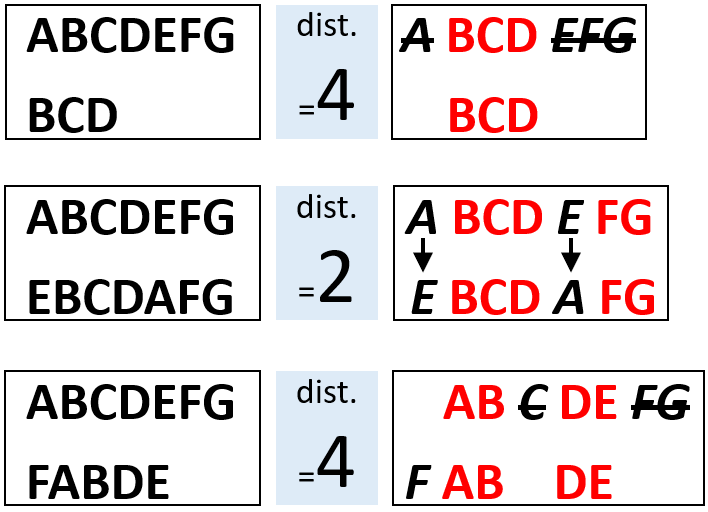Levenstein Ratio (LR) is further defined as follows. Notice that it takes both LD and the length of two strings themselves into consideration, which is quite reasonable. For example, the LD between ‘AB’ and ‘A’ is 1 (one removal operation of ‘B’ at the end), and the LD between ‘ABCDEFGHIJKLMNOPQ’ and ‘ABCDEFGHIJKLMNOP’ is also 1 (one removal operation of ‘Q’ at the end), however, the length of the 2nd group of routes are quite longer, thus a LD of 1 sounds trivial. Therefore, the routes in the 2nd group are more similar with each other than the 1st group. When calculating LR, the 1st group is 0.67, the 2nd group is 0.97, that’s exactly what we expect. LR is ranged between 0 and 1. Higher LR suggests larger similarity.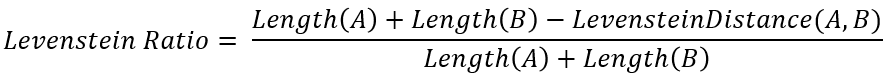When a place is represented as a character, then a route becomes a ordered string. For instance, a string of ‘ABC’ refers to a route of “Place A → Place B → Place C “. Consequently, LR could be generalized to measure the similarity of two routes. For totally N routes, we could get a N*N matrix of pairwise similarities, that is Levenstein Ratio matrix, or saying, similarity matrix.

(2) 路径聚类 Clustering of Routes

This tool apply Affinity Propagation (AP) algorithm to run clustering analysis for routes.

AP聚类的优势是直接基于数据之间的相似度，而是原始数据本身，因此对数据结构没有要求。显然，路径数据是典型的非结构化数据，不能直接适用于经典的K-means等算法，而用AP则正合适。另一方面，AP聚类的输出结果是“原型”，每个原型对应一个类别，认为类别中的数据可以被原型所代表。 因此，AP聚类所找到的原型就是典型路径。
The greatest advantage of AP is no assumption upon the data structure, because it is based on similarity among raw data, instead of raw data itself. Apparently, routes are not structured data,  thus we can not directly use some classical algorithms, such as K-means, at this time, AP can save us. In addition, the results of AP is a set of so-called ‘exemplars’. Each exemplar corresponds to a cluster, and all cases in that cluster are believed to be represented by that exemplar. In this application, the exemplars found by AP could be viewed as typical routes.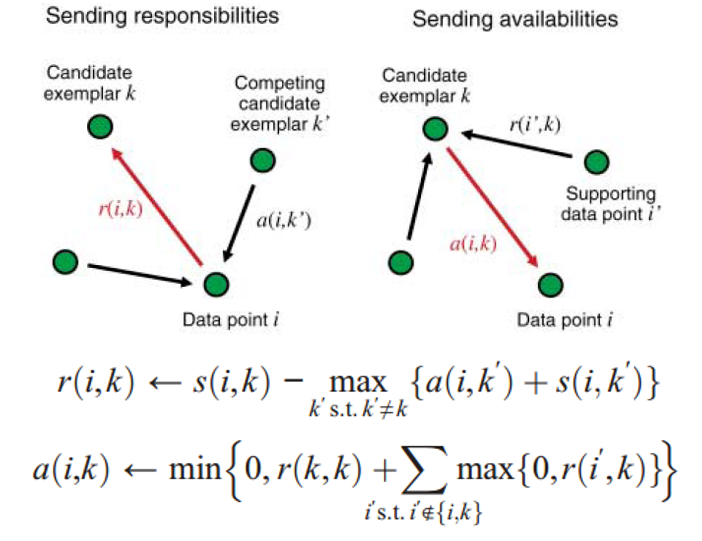AP聚类的基本原理如上图，反映了各个数据点之间传播相似度的过程。其中，r(i,k)是i向k传播的responsibility，可以理解为i希望自己被k（而不是图中其他竞争性的潜在原型k’）代表的程度，a(i,k)是k向i传播的availability，可以理解为k希望作为原型代表i的信心，这种信心来自图中其他数据点i’的支撑。
The basic principle of AP is as above, which shows the process of affinity propagation among data points. r(i,k) is the responsibility propagated from i to k, it is some kind of degree that i hopes it could be represented by k (instead of other competing candidate exemplar k’); and a(i,k) is the availability propagated from k to i, it is some kind of confidence that k holds from other supporting points i’ to be a exemplar to represent i.

AP聚类的主要参数是各个数据点的偏好值，代表了研究者认为（或希望）该点能够成为原型的程度，偏好值越高的点越有可能成为原型。另一方面，偏好值的大小决定了类别数量，偏好值越大，就好像大家都在抢着当原型一样，会得到相对较多的类别数；反之，偏好值越小，就好像大家都不想当原型，而希望被别的点代表，会得到相对较少的类别数。在默认设置中，所有点的偏好相同，均等于相似度矩阵的中位数。AP聚类会根据偏好值设定自动估计出类别数（即典型路径的数量），但个人感觉，默认设置下这个数量经常会显得过多。Matlab中可以指定类别数，再进行聚类，python中不可以，只能人工改变偏好后反复尝试。如果想设定明确的类别数，可以在本工具中导出相似度矩阵，然后再在Matlab中运行AP聚类。
The key parameter of AP is preference of each data point, which representing the degree that researchers believe (or hope) a data point would be a exemplar. The higher the preference, the more likely that point would be a exemplar. In addition, preferences also influence the number of clusters in an interesting way. When we use larger preferences, it would sound like all points are competing to become exemplars, and we will get relatively more number of cluster; in contrast, if we use smaller preferences, it would sound like each point is trying to escape from being chosen as an exemplar, and we will get relatively small number of cluster. By default, preferences of all points are set to a single value, the median of similarity matrix, so we have no assumption upon which point should be given priority to become a exemplar. AP would derive the number of clusters (i.e. the number of typical routes) based on preferences automatically, however, this number seems to be too large in many cases. We can preset the number of clusters in Matlab, but it is not available in python, and the only way to change the number of clusters is to change preferences manually. As to this problem, we could export the similarity matrix from this tool, and then use Matlab to deal with it.

For more technical details, refer to the following paper.

B J Frey, D Dueck. Clustering by passing messages between data points[J]. Science, 2007, 315: 972-976.

(3) 场所在网络中的核心度 Coreness of a place in the network

Coreness is a classical index of core/periphery analysis in social network analysis. In order to use it, we need to build a network of places at first. If an individual goes to both place A and place B, then the association of A and B increase for 1 unit. By this way, we could set a Co-occurrence matrix for all places, the element of which represents the number of individuals who go to both the place of the row and the place of the column. This matrix define the network connections.

In core/periphery analysis, the association of A and B is assumed to be proportional to the coreness of A and B. This idea is quite similar to gravity model without considering spatial impedance. The role of coreness is just like the scale/attractiveness in gravity model. According to this assumption, we could obtain a expected association (co-occurrence) matrix, and we have already got the observed association (co-occurrence) matrix. The most appropriate values of coreness would make these two matrices as similar as possible, as shown below.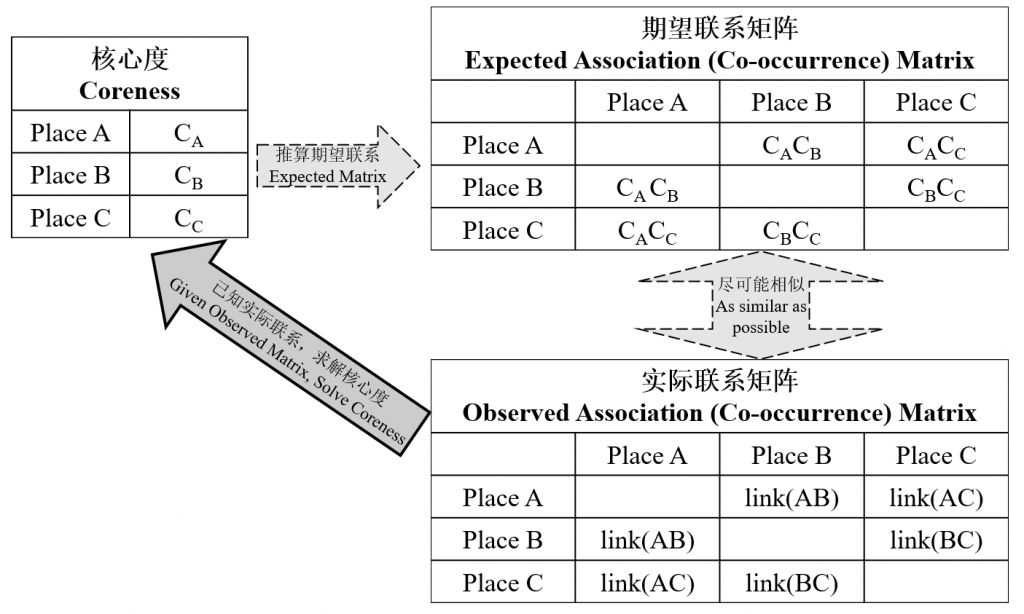We are using gradient descent algorithm to gradually decrease the mean square error between observed and expected association matrices. According to convention, standardized coreness would have a norm of 1.

After the gradient descent algorithm converges, the correlation coefficient between observed and expected association matrices would be reported. A large correlation coefficient suggests that the assumption of ‘Link(AB) = K * Coreness(A) * Coreness(B)’ is reasonable. The higher the coreness of a place, the more important it would be in the place network, because the association of any place with it would be multiplied with a large value.

After we get the coreness of all N places, the algorithm would recommend K places with the largest coreness as core places. The way of recommendation is as follows.  Assuming the number of core places is n, we could set a new theoretically binary coreness vector, which has values of 1 for the n places with the largest coreness, and 0 otherwise. The correlation coefficient of this  vector and the real-value coreness vector could be calculated. Changing n from 0 (no core places) to N (all places are core places), this correlation coefficient would rise at first (too few core places), and then drop down (too many core places). When it reaches the peak value, we get our K.

As to more details of coreness (and core/periphery analysis), please refer to the paper below. Personally, I believe that coreness is better than centrality for real-value (instead of 0/1) network.

S. P. & Everett M. G. Modiels of core/periphery structures[J]. Social Networks, 1999, (21): 375-395.

(4) Apriori

Apriori是经典的关联规则算法，在国内常用于解决著名的啤酒尿布问题——虽然据说这是一个伪命题。Apriori的主要任务是从数据中提取形如“A→B”（或：“如果A，则B”）的关联规则，在本应用可解释为“去过A，则很可能也去B”，其中A可以是多个场所的集合（A1, A2,…），而B只能是单个场所。这种规则实际上一符合一定标准的频繁项集，而最常见的标准是两个——置信度(Confidence)与支持度(Support)。
Apriori is a classical association rule algorithm, which is often mentioned in solving the so-called problem of beer and diaper. Apriori is to extract association rules like ‘A→B’ (or, ‘if A, then B’), in the context of this application, such rules could be explained as “if someone visits place A, he tends to also visit place B”. A could be a set of multiple places (A1, A2, …), while B must be a single place. These rules are actually frequent item-sets that meet certain criteria. The 2 most frequently used criteria are Support and Confidence.

The confidence of a rule ‘A→B’ is the conditional probability of people visiting place B given that they visit place A, or saying P(B|A); and the support is the joint probability of people visiting both A and B, or saying P(AB). For instance, if 20 of 100 individuals have visited placed place A, and 10 of them have also visited place B, then the confidence of the rule “A→B” is P(B|A)=10/20=0.5, the support is P(AB)=10/100=0.1. We should set the thresholds for both confidence and support. When the support of a rule is too low, even the confidence is high enough, it might be just attribute to chance; on the other hand, when the confidence of a rule is too low, we can not judge the direction of the rule.

Another advanced criteria is Lift. As mentioned before, ‘A→B’ should have a high enough confidence P(B|A) to become a rule. However, if we observe a high P(B) without knowing whether or not individuals visit A, then ‘visiting A’ would be not important for ‘visiting B’. For instance, there are three routes “A→B→C”、“A→C”，“B→C”, then  P(B)=2/3=0.67, and P(B|A)=1/2=0.5, which is even less than P(B). At this time, if we want to recommend B to a person, it is not necessary to aim at those who visit A and expect they are more likely to visit B, since it would be even better to recommend B to a random person. Consequently, even though we lower the threshold of confidence to 0.5, ‘A→B’ should not be a rule, because there is not lift for the probability of visiting B given the information of visiting A. The lift of a rule ‘A→B’ is defined as P(B|A) / P(B),  and generally we hope it should larger than 1 for a satisfactory rule.

For more technical details of Apriori , please refer to the following paper.

Agrawal R, Srikant R. Fast algorithms for mining association rules[C]//Proc. 20th int. conf. very large data bases, VLDB. 1994, 1215: 487-499.

(5) 利用循环神经网络预测路径 Predicting routes using recurrent neural network

Recurrent neural network (RNN) is mainly used for predicting sequence data, so it is suitable for predicting routes. Each time, the RNN unit takes the input of the current place of an individual, and outputs his/her choice for the next place. There is a hidden layer connecting the input and output layers, and hidden layers are connected to themselves across time. In another word, the output of the hidden layer at time t (ht) depends not only on the current input (xt), but also the output of the hidden layer at the previous time (ht-1). For example, “…→A→B→C→…” is a piece of a route, and the times for visiting A, B, and C are t1, t2, t3 respectively, then the network structure of RNN is as follows, where wxh,why, whh are weights connecting input and hidden layers, weights connecting hidden and output layers, and weights connecting previous and current hidden layers, f and g are nonlinear activation functions.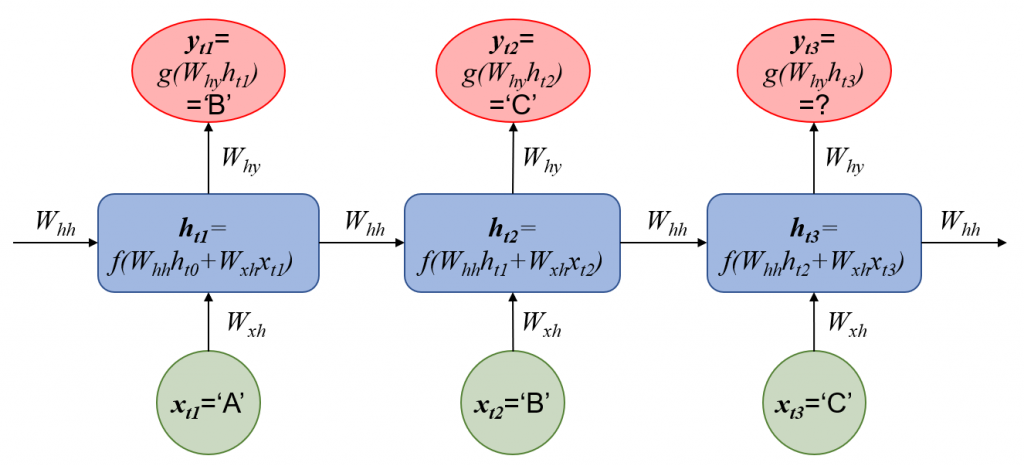The input of this RNN do not consider the features describing the physical environment (such as distance, areas, etc.) of alternative places for the next step, and only use the dummy variable (or saying, one hot encodes) of the current place. So this RNN is actually predicting the next place just according to preview places. which is similar to language model. As the result, it can not predict routes when physical environment changes, and is just for deepening our understanding of current routes. It is certainly possible to introduce these features into neural networks, but we did not make it a general tool for the difficulty of setting different features in different applications.

(6) 集束搜索与最大概率路径 Beam search for the largest probability route

A RNN allows us to calculate the probability of a route and generate a route by continuously predicting the next destination. We would like to find a route with the largest probability, and view it as a typical route. Now the problem is how to choose a destination according to the probability distribution outputted by RNN at each step, so that the final route would has the largest probability?

The most straightforward logic is to choose the place with the largest probability at each step, and this way is called Greedy Search. However, in many cases greedy search would miss the largest probability route, because there might be a place whose probability is not the largest, but having better successors. Beam Search could well solve this problem, it keeps K routes with the largest probabilities  at each step (note: probabilities for the current routes, not for the destinations at that single step) for searching at the next step.

For example, there are totally 45 places as alternatives, as shown in the following figure, and we set K=3. At the first step, the 3 places with the largest probabilities are 1, 2, 3. Assuming we choose place 1 at the first step, so we have to calculate the probabilities of routes ‘1→1’, ‘1→2, ‘…, ‘1→45’; assuming we choose place 2 at the first step, so we will also calculate the probabilities of routes ‘2→1’, ‘2→2’, …, ‘2→45’; similarly,, we would also calculate the probabilities of routes ‘3→1’, ‘3→2’,…, ‘3→45’. As the result, there would be totally 3*45 = 135 routes, from which we would choose 3 routes with the largest probabilities, ‘3→43’, ‘3→1’, ‘1→3’. Now let’s move to the third step. Assuming we have chosen ‘3→43’ at the first 2 steps, we will calculate the probabilities of routes ‘3→43→1’, ‘3→43→2’, …, ‘3→43→45’; assuming we have chosen ‘3→1’ at the first 2 steps, we would calculate the probabilities of routes ‘3→1→1’, ‘3→1→2′,…’3→1→45’; similarly, we would also calculate the probabilities of routes ‘1→3→1’, ‘1→3→2’,…, ‘1→3→45’. Again, there would be totally 3*45=135 routes, from which we would choose 3 routes with the largest probabilities. At this time, the route with the largest probability is already ‘3→1→2’, not ‘3→43→#’. If we apply greedy search, we would choose place 43 at the second step, and thus miss this route.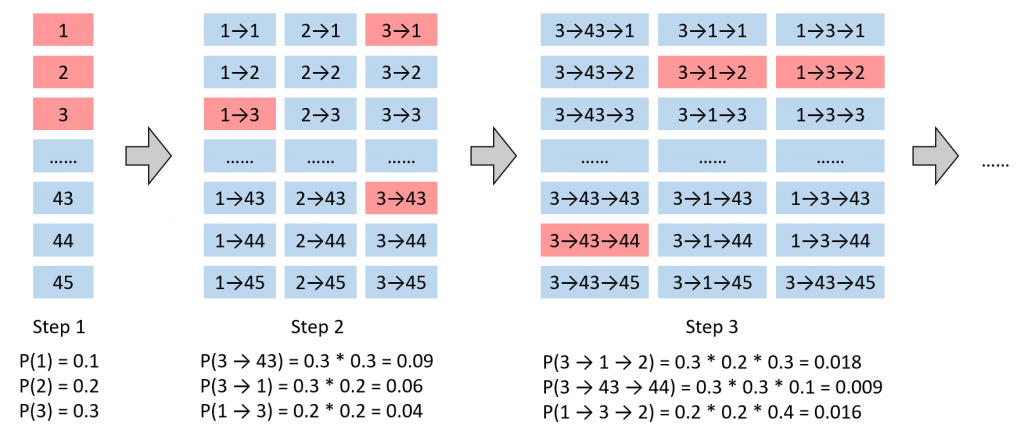K是集束搜索的唯一参数。K越大，集束搜索得到的路径真的是概率最大路径的信心越强，但相应的计算成本也更高，反之亦然。当K=1时，集束搜索退化为贪婪搜索。
K is the only parameter of beam search. A larger K would give more confidence that the route outputted by beam search would really has the largest probability, however, it would more computational expensive, vice versa. When K=1, beam search is just the same as greedy search.

ICP备案号：沪ICP备20010289号-1